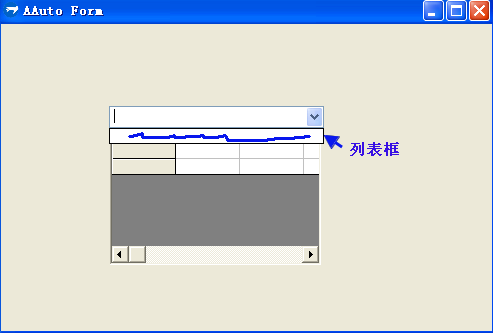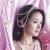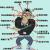快手 combobox 问题

年薪达不到25W大数据工程师、拿不到Offer全额退款->>>import win.ui;
/*DSG{{*/
var winform = ..win.form(text="AAuto Form";right=490;bottom=306)
combobox={cls="combobox";text="combobox";left=108;top=82;right=323;bottom=102;edge=1;items={};mode="dropdown";z=1};
static={cls="static";text="static";left=108;top=84;right=321;bottom=242;hide=1;transparent=1;z=2}
)
/*}}*/

import com;
var dataGrid = winform.static.createEmbed("MSFlexGridLib.MSFlexGrid.1");
var gd = dataGrid._object

// 初始化界面
with gd{
Cols =4 // 列
Rows = 4 //行
setTextMatrix(0,0,"材料编码")
setTextMatrix(0,1,"材料名称")
setTextMatrix(0,2,"规格")
setTextMatrix(0,3,"单位")
}

winform.combobox.oncommand = function(id,event){
if(event == 0x7/*_CBN_DROPDOWN*/){
winform.static.hide = 0
}
}

winform.show()
win.loopMessage();0import win.ui;
/*DSG{{*/
var winform = ..win.form(text="AAuto Form";right=490;bottom=306)
combobox={cls="combobox";text="combobox";left=108;top=82;right=323;bottom=102;edge=1;items={};mode="dropdown";z=1};
static={cls="static";text="static";left=105;top=103;right=318;bottom=261;hide=1;notify=1;transparent=1;z=2}
)
/*}}*/

import win.subclass
import com;
var dataGrid = winform.static.createEmbed("MSFlexGridLib.MSFlexGrid.1");
var gd = dataGrid._object

// 初始化界面
with gd{
Cols =4 // 列
Rows = 4 //行
setTextMatrix(0,0,"材料编码")
setTextMatrix(0,1,"材料名称")
setTextMatrix(0,2,"规格")
setTextMatrix(0,3,"单位")
}

winform.combobox.oncommand = function(id,event){
if(event == 0x7/*_CBN_DROPDOWN*/){
::PostMessage(winform.combobox.hwnd, 0x14F/*_CB_SHOWDROPDOWN*/, 0, 0);
winform.static.hide = 0
}
}

winform.show()
win.loopMessage();

00